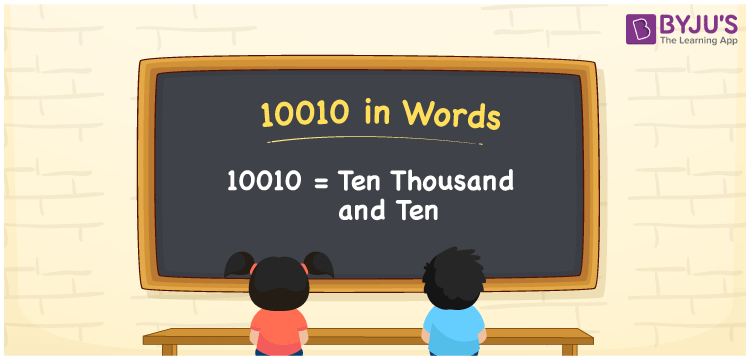# 10010 in Words

10010 in words is written as “Ten Thousand and Ten”. In Maths, 10010 is a natural number, that is used to represent a value or a count. Hence, 10010 is given by Ten Thousand and Ten in English. In real life also, we use numbers in words to express a value. For example, Rs.10010 is expressed as “Rupees Ten Thousand and Ten only” in a cheque.

 10010 in Words Ten Thousand and Ten Ten Thousand and Ten in Numerical Form 10010

## 10010 in English Words## How to Write 10010 in Words?

We can convert 10010 to words using a place value chart, that represents the positions of digits in a number. The number 10010 has 5 digits, hence, the digits are represented as:

 Ten thousand Thousands Hundreds Tens Ones 1 0 0 1 0

We can see, from the above table,

1 → Ten Thousands

0 → Thousands

0 → Hundreds

1 → Tens

0 → Ones

Now, read the digits from right to left. Thus, together we can write the number as Ten Thousand and Ten.

### Expanded Form of 10010

We can write the expanded form as:

1 × Ten thousand + 0 × Thousand + 0 × Hundred + 1 × Ten + 0 × One

= 1 × 10000 + 0 × 1000 + 0 × 100 + 1 × 10 + 0 × 1

= 10010

= Ten Thousand and Ten

10010 is the natural number that is succeeded by 10009 and preceded by 10011. Learn more about the number 10010 below:

• 10010 in Words – Ten Thousand and Ten
• Is 10010 an odd number? – No
• Is 10010 an even number? – Yes
• Is 10010 a perfect square number? – No
• Is 10010 a perfect cube number? – No
• Is 10010 a prime number? – No
• Is 10010 a composite number? – Yes

## Frequently Asked Questions on 10010 in words

Q1

### What is 10010 in words?

10010 in words is given by Ten Thousand and Ten.
Q2

### What is the 10010 minus 10?

Ten thousand and ten minus ten is equal to Ten thousand.
Q3

### Is 10010 a perfect square?

No, 10010 is not a perfect square because it can be represented as the product of two same integers.
Test your Knowledge on 10010 in Words